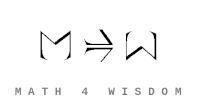文章 发现 ms@ms.lt +370 607 27 665 My work is in the Public Domain for all to share freely. 读物 书 影片 维基百科 Software Upload How do derived functors express the threesome? 導出函子 Understand how derived functors relate the three-cycle and the foursome. Understand how derived functors relate the four functors on sheaves to the foursome Understand how setting {$R^2F=0$} yields the gradation and the eightfold way. What does it mean to compute the cohomology at the {$i$}th spot? This yields {$RF$}. 读物 维基百科: Derived functor A circulation - a three-cycle - is a self-adjoint functor which constructs itself as it goes along.
Šis puslapis paskutinį kartą keistas March 07, 2021, at 10:21 AM